# Planimetrics - math word problems

Study plane measurements, including angles, distances, and areas. In other words - measurement and calculation of shapes in the plane. Perimeter and area of plane shapes.

#### Number of problems found: 1973

• The Eiffel Tower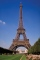The top of the Eiffel Tower is seen from a distance of 600 meters at an angle of 30 degrees. Find the tower height.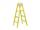The double ladder has 3 meters long shoulders. What is the height of the upper of the ladder reach if the lower ends are 1.8 meters apart?
• The bridge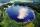Across the circle lakepasses through its center bridge over the lake. At three different locations on the lake shore are three fishermen A, B, C. Which of fishermen see the bridge under the largest angle?
• Tiles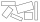The tile has the shape of a square with a side of 15 cm. What dimensions can a rectangle composed of 90 of these tiles have so that no tile remains?How long is a ladder that touches on a wall 4 meters high and its lower part is 3 meters away from the wall?
• FloorRectangular floor of living room has a length 5.4 meters and a circumference 17.2 meters. What is its width?
• Goat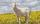Meadow is a circle with radius r = 19 m. How long must a rope to tie a goat to the pin on the perimeter of the meadow to allow goat eat half of meadow?
• Reservoir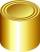6 m long reservoir has a diameter of 2.2 m. What is its surface area in square meters?
• Central angleWhat is the length of the arc of a circle with a diameter of 46 cm, which belongs to a central angle of 30°?
• Cylinder containerThe cylindrical container with a diameter of 1.8 m contains 2,000 liters of water. How high does the water reach?
• Space diagonalThe space diagonal of a cube is 129.91 mm. Find the lateral area, surface area and the volume of the cube.
• The angle of viewDetermine the angle of view at which the observer sees a rod 16 m long when it is 18 m from one end and 27 m from the other.
• Diagonals of a rhombusOne of the diagonals of a rhombus is twice as the other. If the sum of the lengths of the diagonals is 24, find the area of the rhombus.
• Isosceles triangleCalculate the area of an isosceles triangle, the base of which measures 16 cm and the arms 10 cm.
• CosineCalculate the cosine of the smallest internal angle in a right-angled triangle with cathetus 3 and 8 and with the hypotenuse 8.544.
• Diamond sideThe diagonals of the diamond are 18 cm and 14 cm long. Calculate the length of the diamond side.
• The parallelogram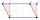The parallelogram has one side 2 dm long, which is one-sixth of its circumference. How many cm does the other side of the parallelogram measure?
• Lake or pondThe landlord has a square lake. Trees grow around this lake. The lake wants to enlarge the pond twice and does not want to cut down or flood any tree. How will he do that?
• Quarter of a circleCalculate the circumference of a quarter circle if its content is S = 314 cm2.
• Map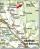Forest has an area of ​​36 ha. How much area is occupied by forest on the map at scale 1:500?

Do you have an interesting mathematical word problem that you can't solve it? Submit a math problem, and we can try to solve it.

We will send a solution to your e-mail address. Solved examples are also published here. Please enter the e-mail correctly and check whether you don't have a full mailbox.

Please do not submit problems from current active competitions such as Mathematical Olympiad, correspondence seminars etc...